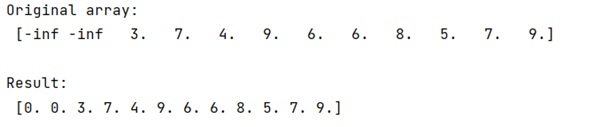# Replace -inf with zero value in NumPy array

Given a NumPy array, we have to replace -inf with zero value in NumPy array.
Submitted by Pranit Sharma, on February 14, 2023

NumPy is an abbreviated form of Numerical Python. It is used for different types of scientific operations in python. Numpy is a vast library in python which is used for almost every kind of scientific or mathematical operation. It is itself an array which is a collection of various methods and functions for processing the arrays.

## NumPy Array - Replace -inf with zero value

Suppose that we are given a numpy array that contains some numerical values along with some -inf. We need to replace -inf with zeroes.

For this purpose, we will use numpy.isneginf() method which is used to return a boolean value as True if the negative infinity value is present. Hence, we will check where -inf is present and assign 0 at that position.

Let us understand with the help of an example,

## Python code to replace -inf with zero value in NumPy array

```# Import numpy
import numpy as np

from numpy import inf

# Creating a numpy array
arr = np.array([-inf, -inf, 3,7,4,9,6,6,8,5,7,9])

# Display original array
print("Original array:\n",arr,"\n")

# replacing -inf with 0
arr[np.isneginf(arr)] = 0

# Display result
print("Result:\n",arr)
```

Output: